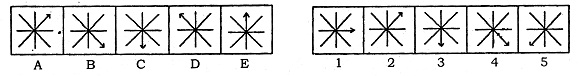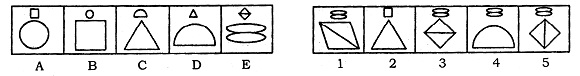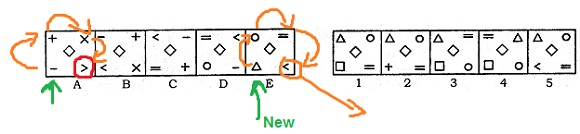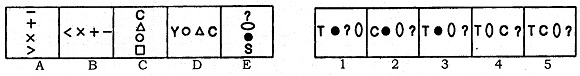# Non - verbal reasoning (Series)

Non-Verbal reasoning appears in Bank exams, Infosys, MAT exams constantly. There are 5 Problem Figures (PF) will be given with 5 Answer Figures (AF).  We need to determine the next figure in the series.  There are certain rules which make solving these problems easy.  So study the rules and solved examples.

Step 1:
For all the series problems the following rules apply.  If problem figures A and E are equal our answer is problem figure B. Similarly, the other rules as follows.

1. PF(A) = PF(E) then answer is PF(B)
2. PF(D) = PF(E) then answer is PF(C)
3. PF(A) = PF(C) = PF(E) then answer is PF(B)
4. PF(A) = PF(D), PF(B) = PF(E) then answer is PF(C)
5. PF(D) = inverse of PF(A) and PF(E) = inverse of PF(2) then answer is inverse of PF(C)

Step 2:
In general, the items in the box takes different positions in the subsequent figures.  They may rotate certain degrees either clock wise or anti-clockwise.  Look at the following diagram. In some problems new items add to the existing figures and some existing figures vanish.Solved Examples

1.In this problem If PF(A) = PF(E) then answer is PF(B).  In the answer options AF(4) is same as PF(B) so option 4

2.Here PF(C) and PF(E) are equal.  So Answer figure should be PF(B).  So correct option is c.

3.The arrow is changing its positions clock wise 90o, 45o, 135o, 45o, ....next should be 180o. So option 3.

4.Simple one.  A new arrow and a new line are adding alternatively.  In PF(E) a new line has added.  So in the next figure a new arrow must be added.  And total lines should be 6.  Option 5

5.Small hand is moving anticlock wise 90o, 45o, 90o, 45o,... and Big hand is moving clock wise 135o constantly.  So in the next figure, small hand must move 90o anti clockwise, and big hand must move 135o.  So option 4

6.Here the symbol is changing positions anti clockwise by  45o and every time a new symbol is adding. The "C"s in the middle are rotating clock wise by 90o. So the next figure must be option 4

7.This is a simple analogy.  There is a relationship between 1 and 2, 3 and 4.  the small figures in the first diagram are getting bigger and vice-versa.  So Option 3

8.All the three symbols in the dice are rotating clockwise.  So option 3

Alternative method:

We know that if  PF(A) = PF(D), PF(B) = PF(E) then answer is PF(C).  So option 3

9.A new symbols is appearing in the middle of the previous figure and the previous figure is getting bigger. So option 4 is the right option.  3 and 5 options are ruled out as the figures in the middle are appeared already.

10.A dot and line are adding constantly to the figures in left and right sides alternatively.  So option 3

11.There appears to be no pattern on immediate look, but his problem can be solved by simple observation.  Have a look at the diagram below..The positions of two symbols are not changing in 2 consecutive figures.  So option 5

12.the arrow and small line inside the small square are rotating constantly anti clockwise and clockwise respectively by 90o, 45o, 90o, 45o,... and 45o, 90o, 45o, 90o .  So next figure would be option 3.

13.The line is rotating anti clock wise by 90o, 180o, 270o, 360o  so next figure should be 90o from figure E and a new symbol must appear.  So option 1 is the correct.

14.Symbols X is rotating clockwise by 45o, 90o, 45o, 90o.  So our options will be either 1 or 3 as in the next figure symbol X must move 45o.  A new symbols is being added to X each time one at front and next time at back.  So option 3 is right one.

15.Simple.  Observe PF(A) and PF(E) are equal.  So next figure will be PF(B).  So option 5

16.the symbols are changing constantly in clockwise direction and a new symbol is being added.The red rounded circle is a place whenever a symbol appear in that position must not appear in the next.  And remaining positions are moving clockwise by 90o.  A new symbol must come at the place shown by green arrow.  So our option will be 1.  Option 2 is ruled out as + symbol appeared earlier.

17.Circle is moving diagonally and triangle is moving clockwise by 90o. So option 1 is correct one.

18.Here you can easily observe that the lines are rotating 90o clockwise. also in PF(B) and PF(D), half line has added at the right most side and in figures PF(C) and PF(E) a new line has added. So in our answer half line has to be added and lines should rotate 90o.  So answer option 2.

19.Simple one.  Figures A and B changed their symbols opposite them.  C and D also did So.  So option 1

20.Symbols in  A, B are same except Symbols at bottom.  A new symbol is coming there.  Similarly in C, D.  So option 3.  Option 2 is ruled out as C appeared earlier.